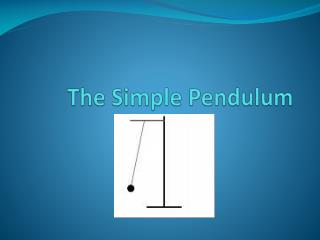DownloadDownload PresentationThe Simple Pendulum

# The Simple Pendulum

Download Presentation## The Simple Pendulum

- - - - - - - - - - - - - - - - - - - - - - - - - - - E N D - - - - - - - - - - - - - - - - - - - - - - - - - - -
##### Presentation Transcript

1. The Simple Pendulum

2. What is a Pendulum? • Is a WEIGHT suspended from a PIVOT so it can swing freely. • When displaced it is subject to a restoring force due to gravity which allows it to accelerate back and forth. • Kinetic vs. Potential • The time for one complete cycle, a left swing and a right swing is called the PERIOD.

3. Building a Pendulum • 1. A String or Rod • 2. A Weight. • 3. Amplitude • 4. Gravity

4. Assumptions • Massless rod • Massive bob • Frictionless pivot • Low theta

5. Pendulums in Technology • Pendulum Clocks -Discovery (Galileo Galilei - 1602) -Pendulum Clock (Christiaan Huygens – 1656) - Error - 15 min per day reduced to 15 sec - Replaced by quartz clock in 1930s ***WHY WAS IT USED FOR TIME KEEPING?

6. Considering the Following..... • In your groups consider the following variables when constructing a pendulum and how or if they affect period. • MASS • LENGTH • AMPLITUDE • GRAVITY Explain Your Reasoning!

7. Formula for Simple Pendulum • T= Period m/s • π= 3.14 • L= length m • g= acceleration due to gravity (9.8m/s²)

8. Example for Finding Period • Ex: Find the period of a pendulum with a string length of 3 meters. • Answer = 3.5 seconds

9. Making the calculations • In your groups you will be asked to find the string length for your given periods. Group 1 T= 1 sec Group 2 T = 1.5 sec Group 3 T = 2 sec Answers: G1 = .25m, G2 =.56m, G3 = 1m